The plot_hist_numeric() to visualizes the histogram of numeric data or relationship to specific categorical data.

plot_hist_numeric(.data, ...)

# S3 method for data.frame
plot_hist_numeric(
.data,
...,
title = "Distribution by numerical variables",
each = FALSE,
typographic = TRUE,
base_family = NULL
)

# S3 method for grouped_df
plot_hist_numeric(
.data,
...,
title = "Distribution by numerical variables",
each = FALSE,
typographic = TRUE,
base_family = NULL
)

## Arguments

.data data.frame or a tbl_df or a grouped_df. one or more unquoted expressions separated by commas. You can treat variable names like they are positions. Positive values select variables; negative values to drop variables. If the first expression is negative, plot_hist_numeric() will automatically start with all variables. These arguments are automatically quoted and evaluated in a context where column names represent column positions. They support unquoting and splicing. character. a main title for the plot. logical. Specifies whether to draw multiple plots on one screen. The default is FALSE, which draws multiple plots on one screen. logical. Whether to apply focuses on typographic elements to ggplot2 visualization. The default is TRUE. if TRUE provides a base theme that focuses on typographic elements using hrbrthemes package. character. The name of the base font family to use for the visualization. If not specified, the font defined in dlookr is applied. (See details)

## Details

The histogram helps determine whether the distribution of a numeric variable. plot_hist_numeric() shows box plots of several numeric variables on one screen. This function can also display a histogram for each level of a specific categorical variable. The bin-width is set to the Freedman-Diaconis rule (2 * IQR(x) / length(x)^(1/3))

The base_family is selected from "Roboto Condensed", "Liberation Sans Narrow", "NanumSquare", "Noto Sans Korean". If you want to use a different font, use it after loading the Google font with import_google_font().

## Examples

# \donttest{
# Visualization of all numerical variables
plot_hist_numeric(heartfailure)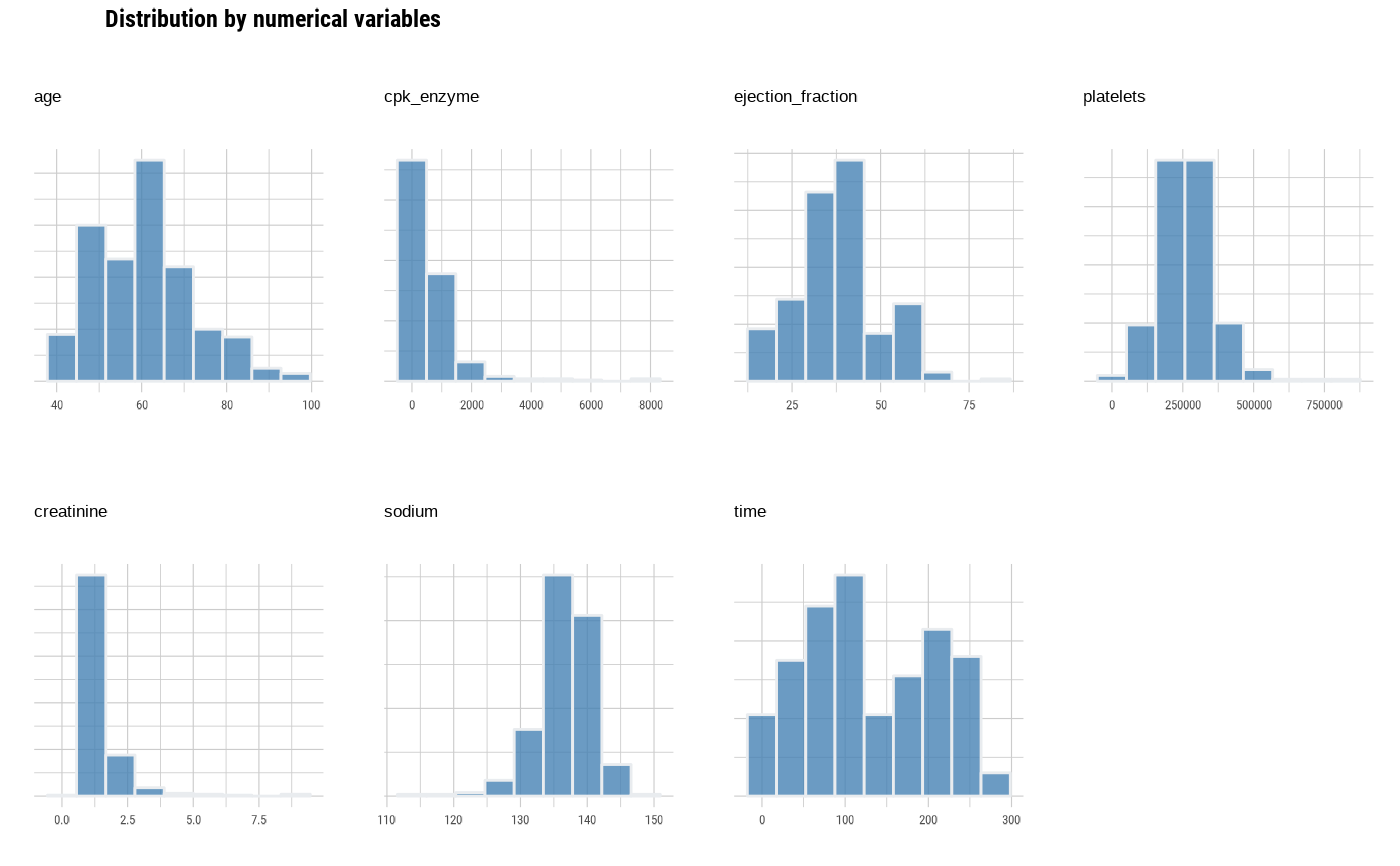# Select the variable to diagnose
plot_hist_numeric(heartfailure, "age", "time")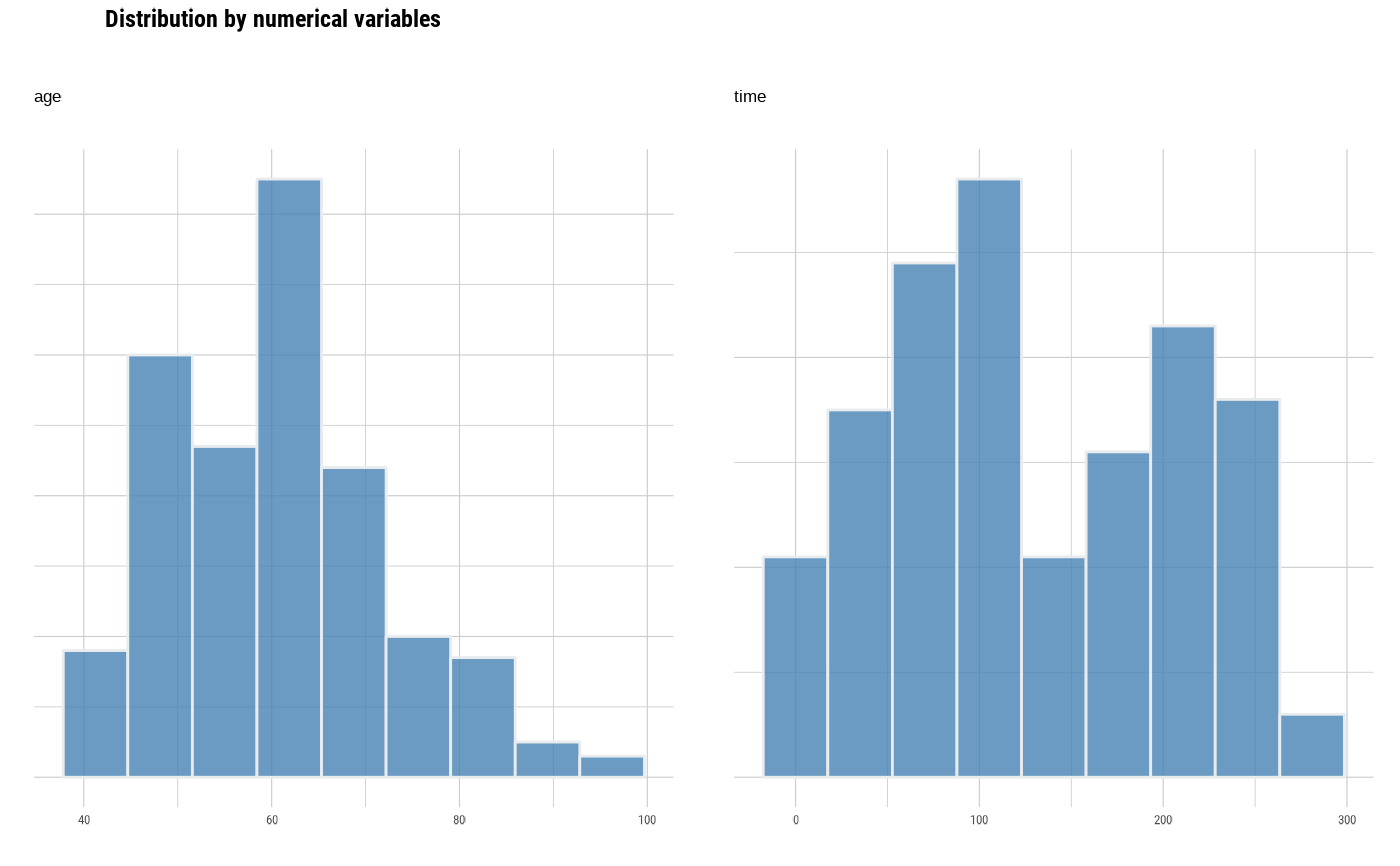plot_hist_numeric(heartfailure, -age, -time)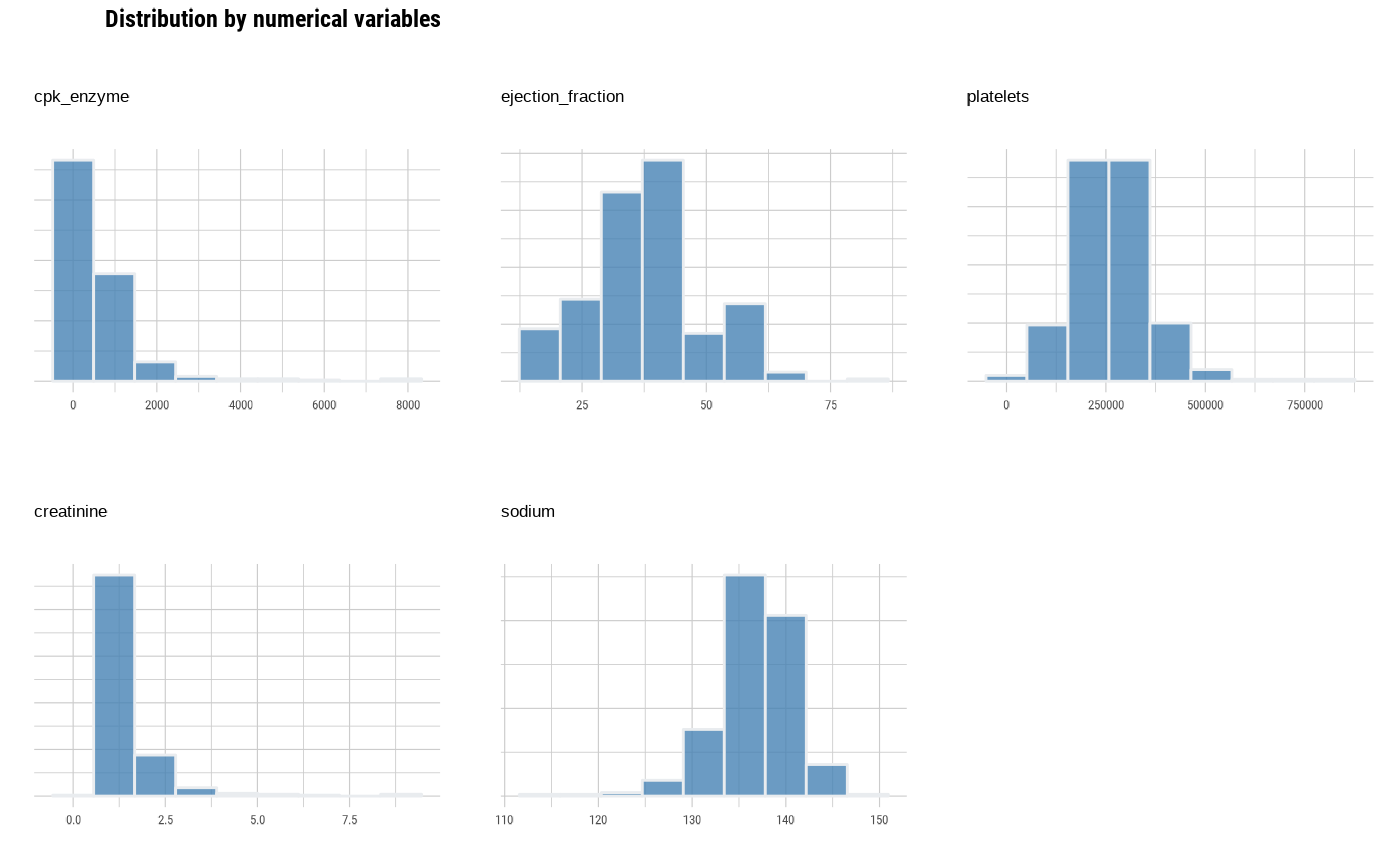# Visualize the each plots
plot_hist_numeric(heartfailure, "age", "time", each = TRUE)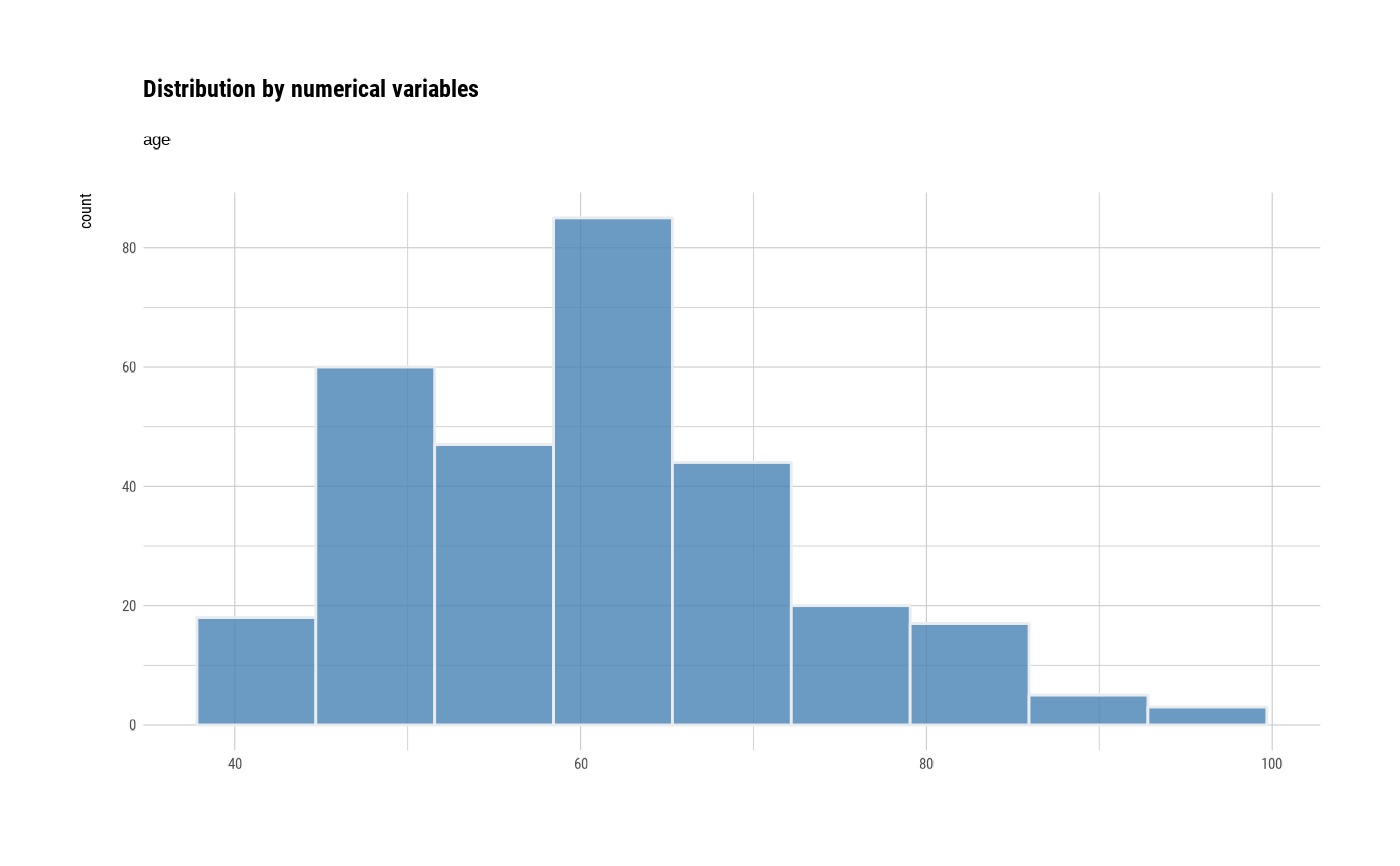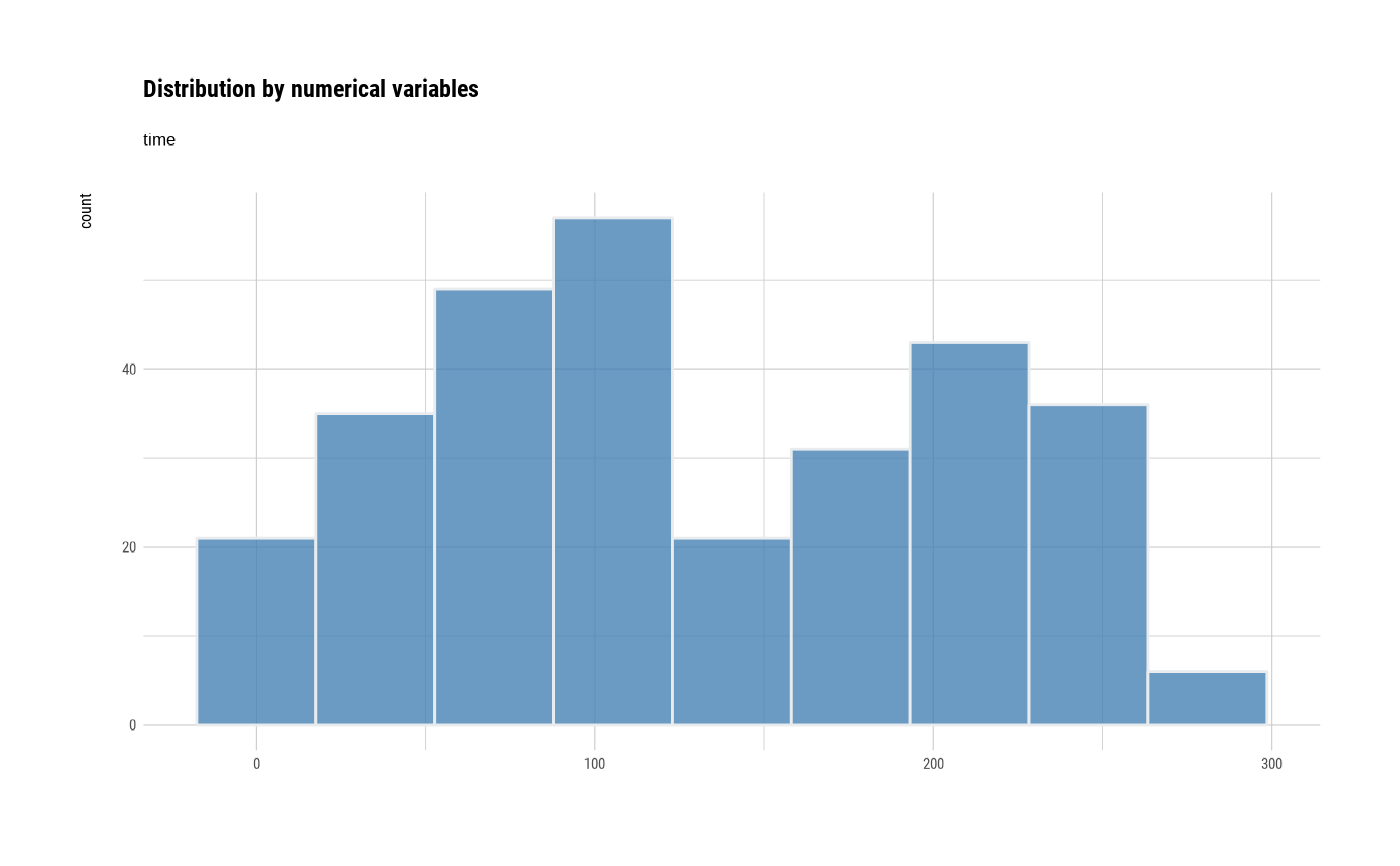# Not allow the typographic elements
plot_hist_numeric(heartfailure, typographic = FALSE)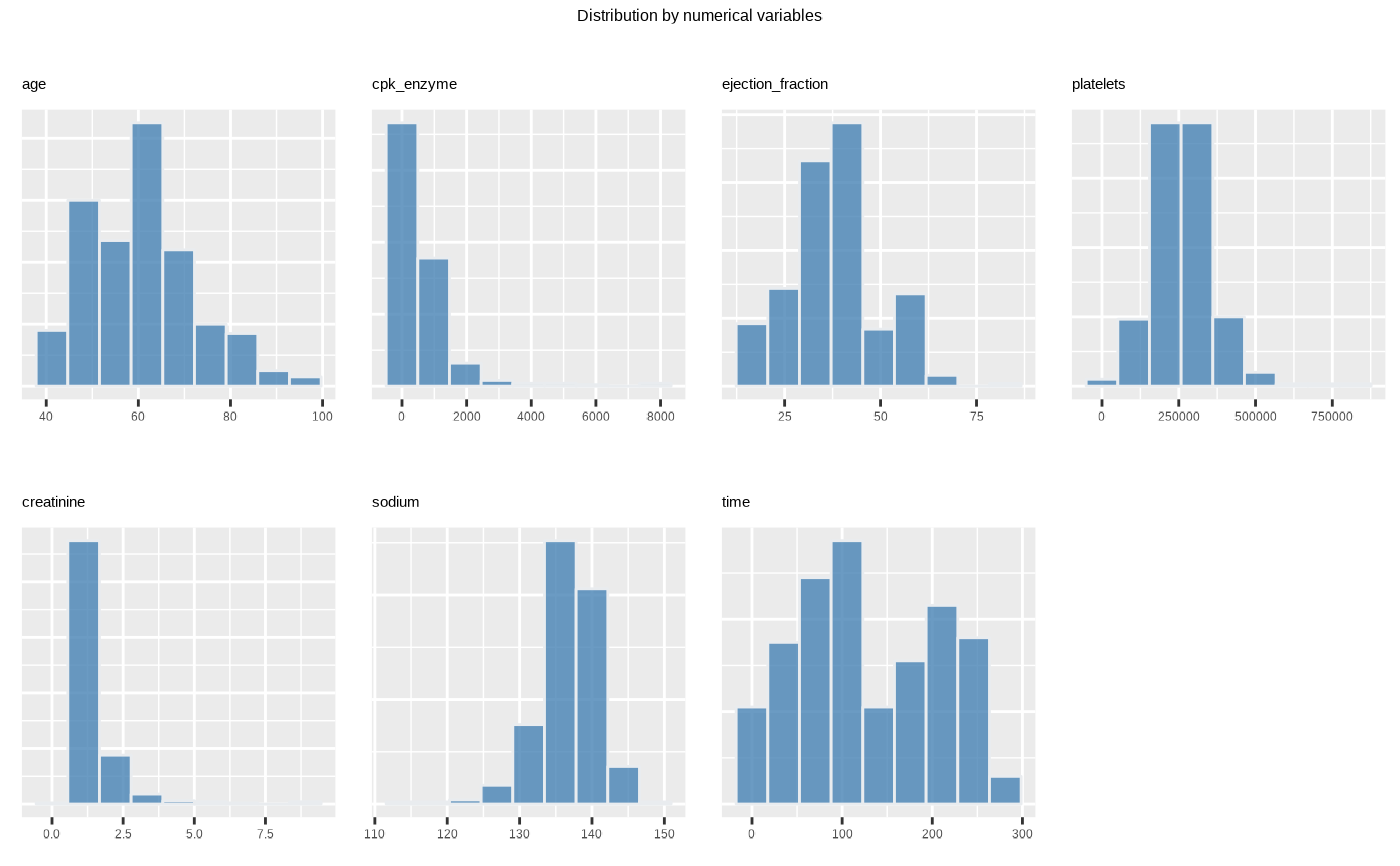# Using pipes ---------------------------------
library(dplyr)

# Plot of all numerical variables
heartfailure %>%
plot_hist_numeric()# Using groupd_df  ------------------------------
heartfailure %>%
group_by(smoking) %>%
plot_hist_numeric()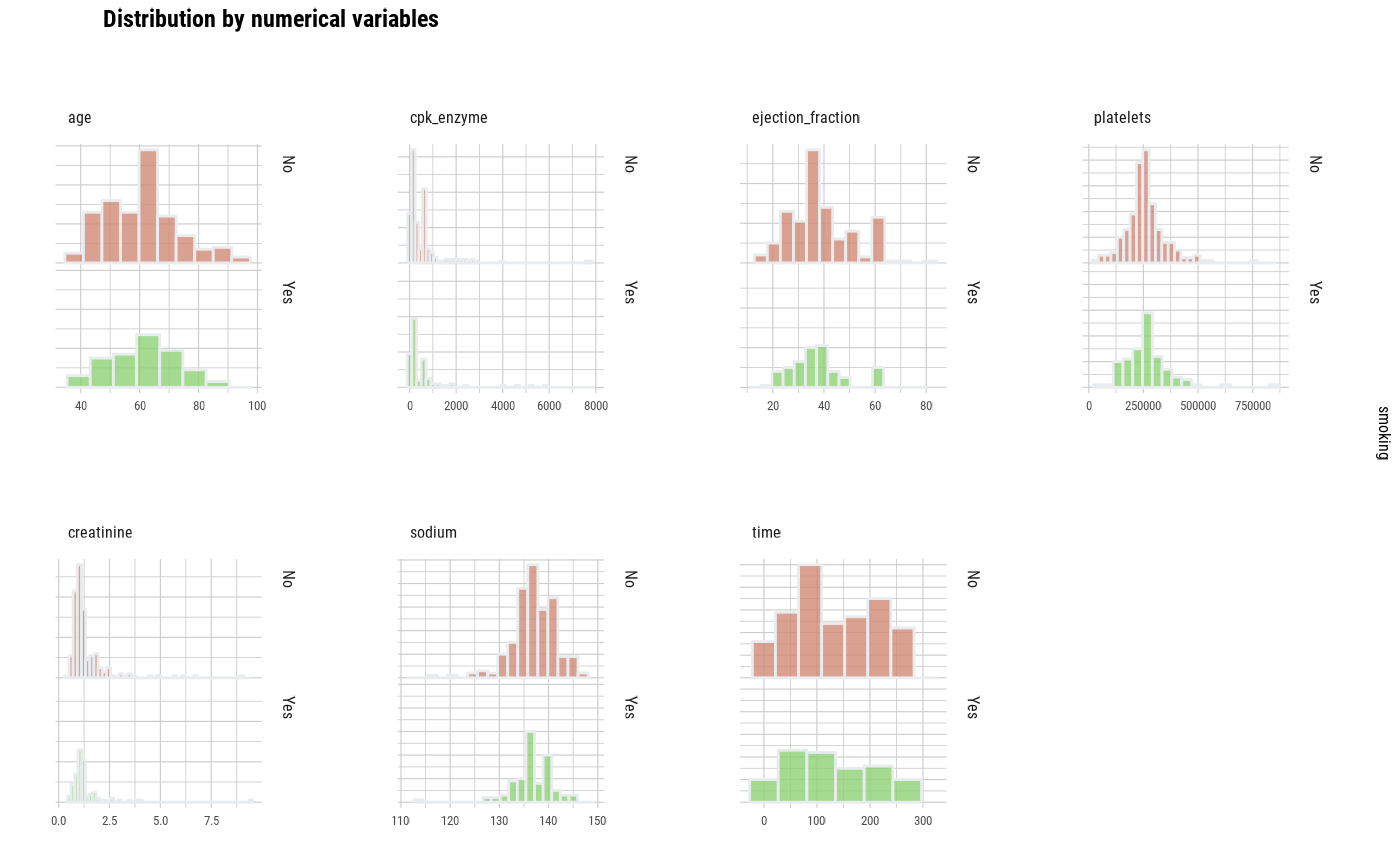heartfailure %>%
group_by(smoking) %>%
plot_hist_numeric(each = TRUE)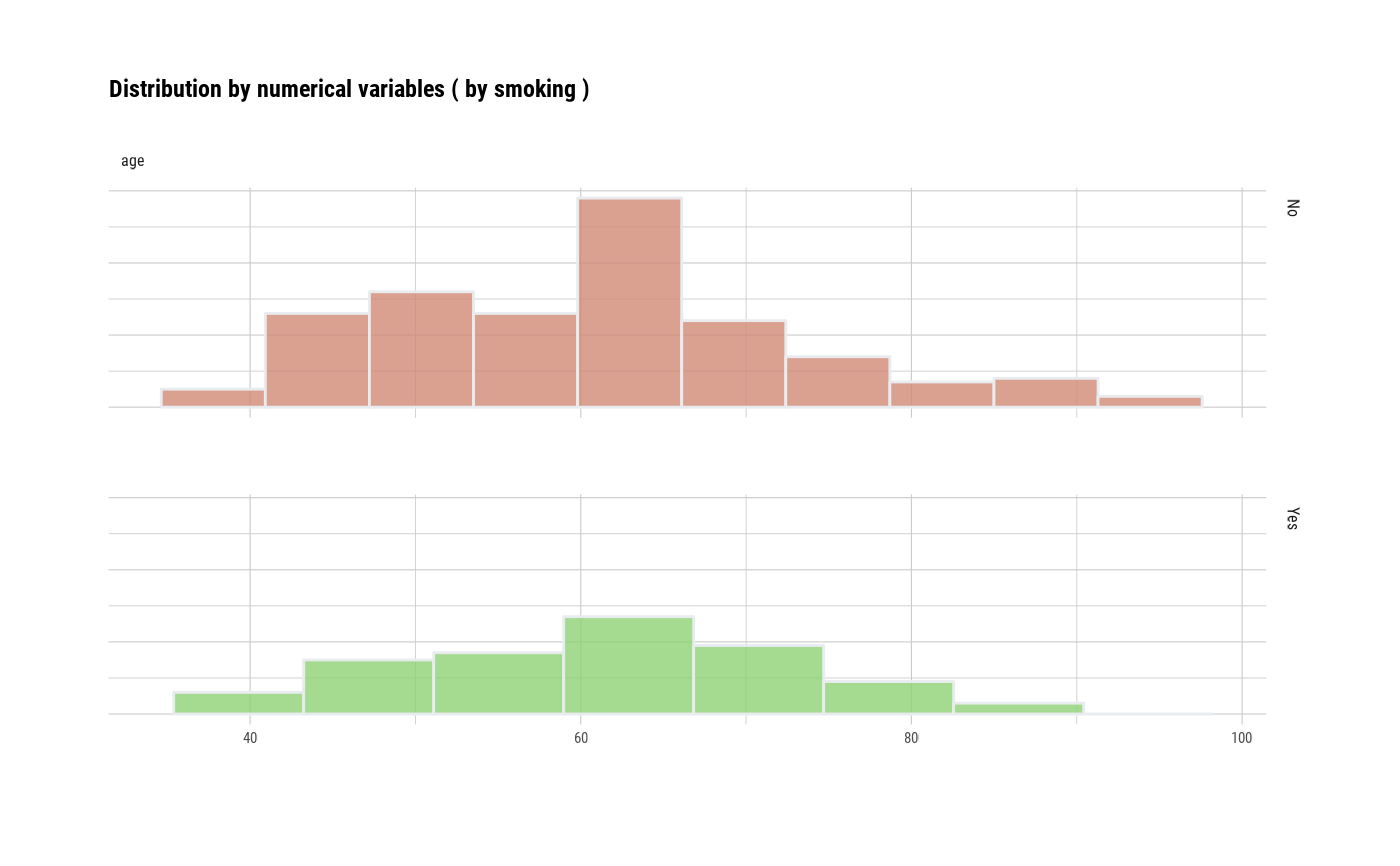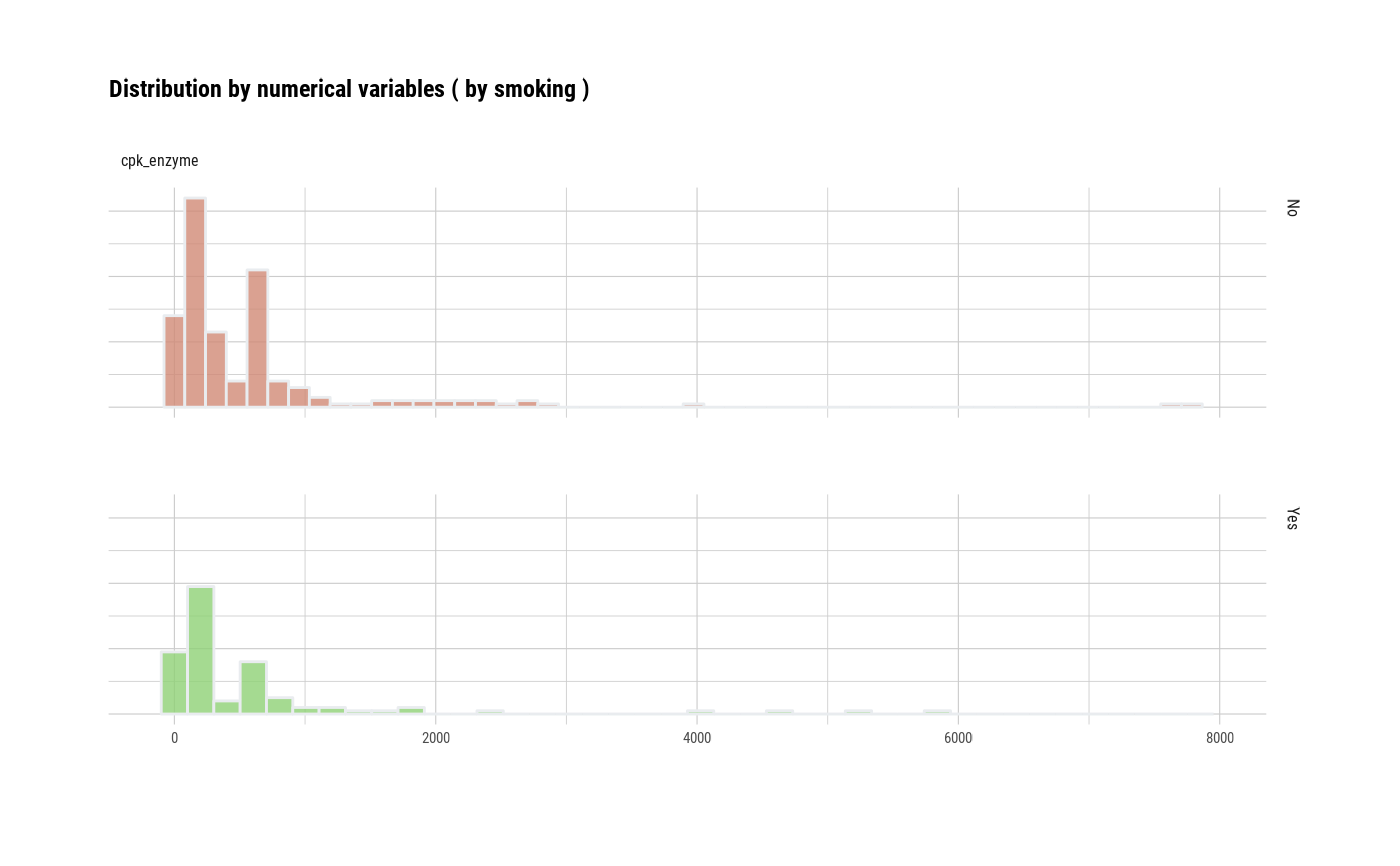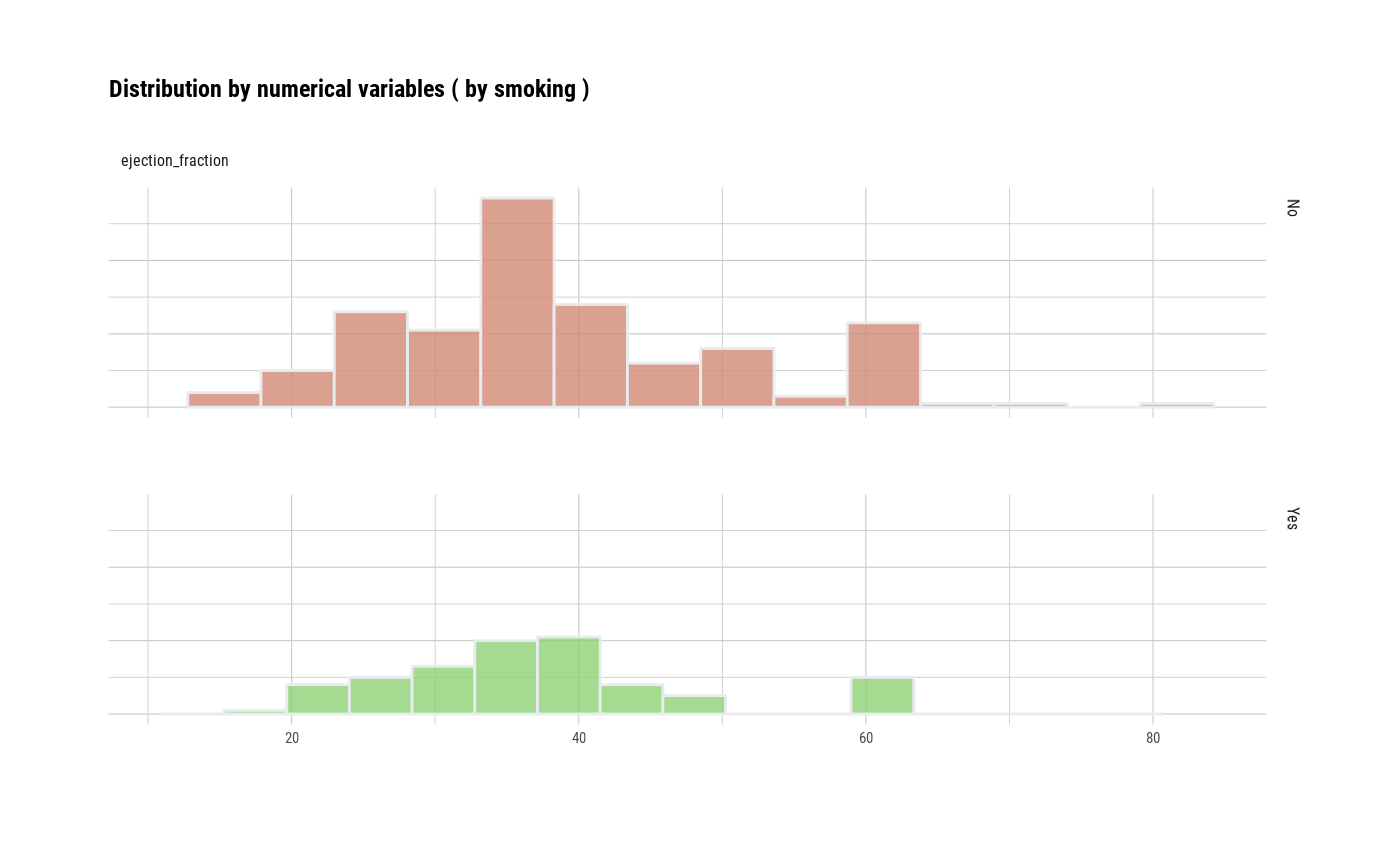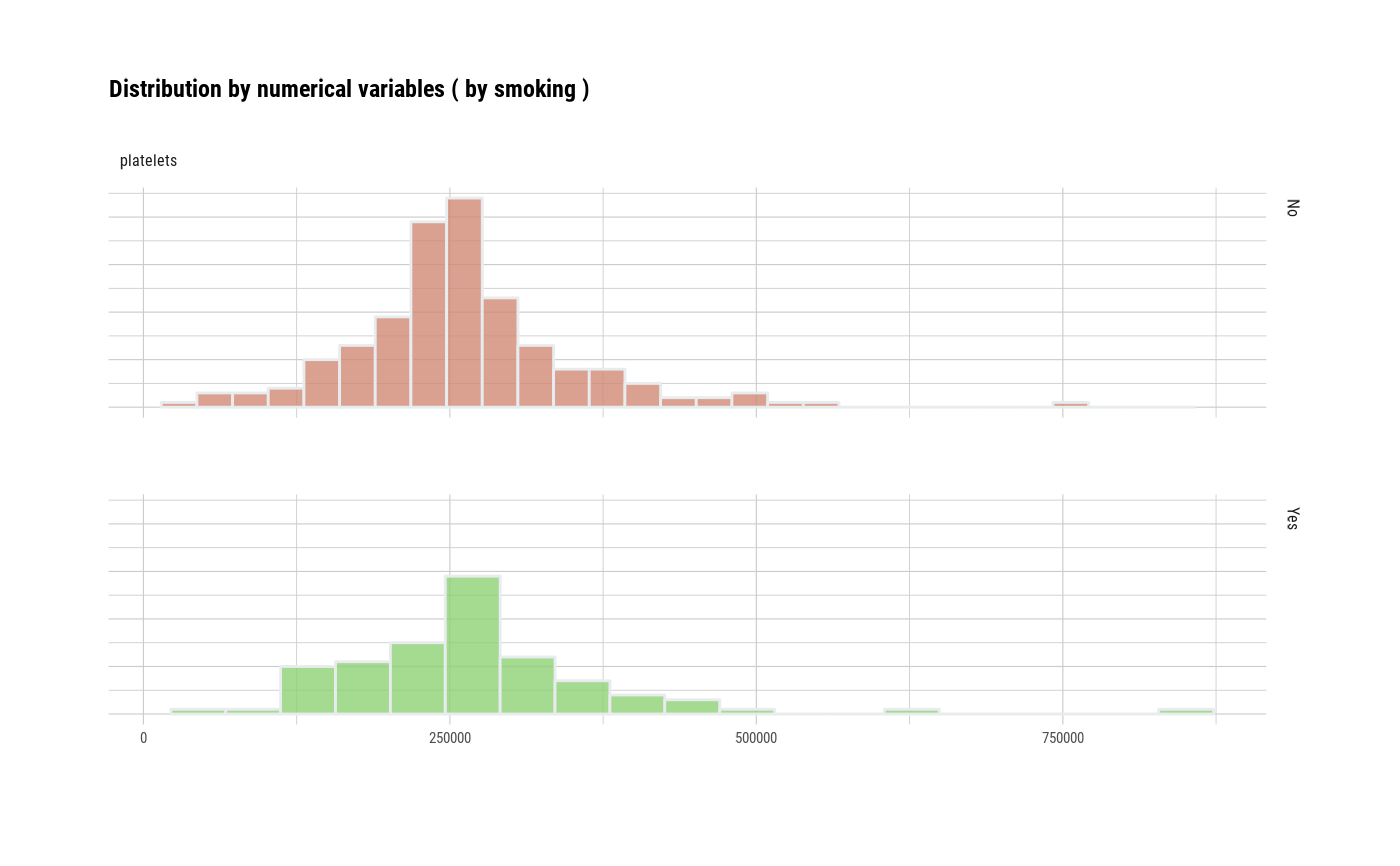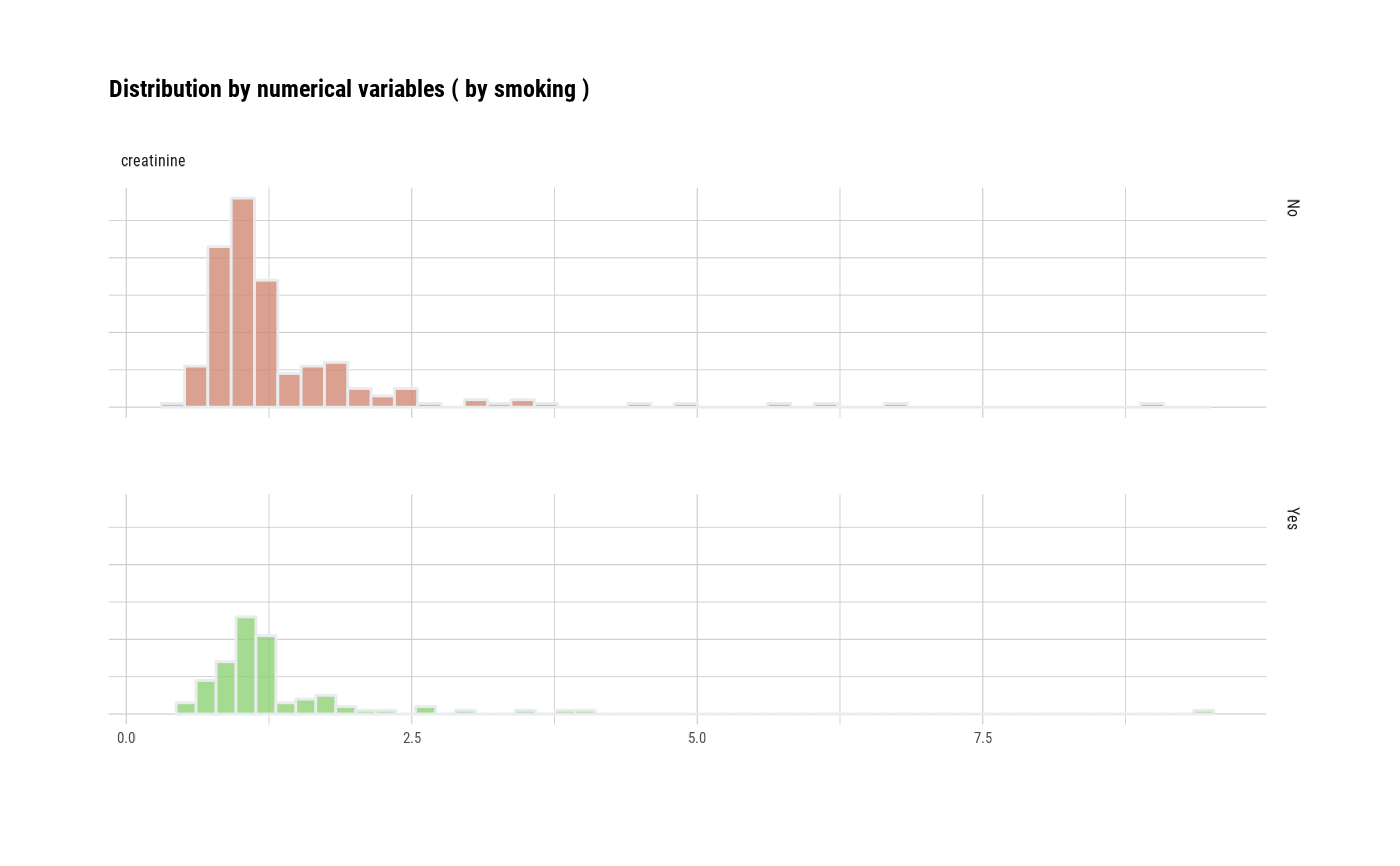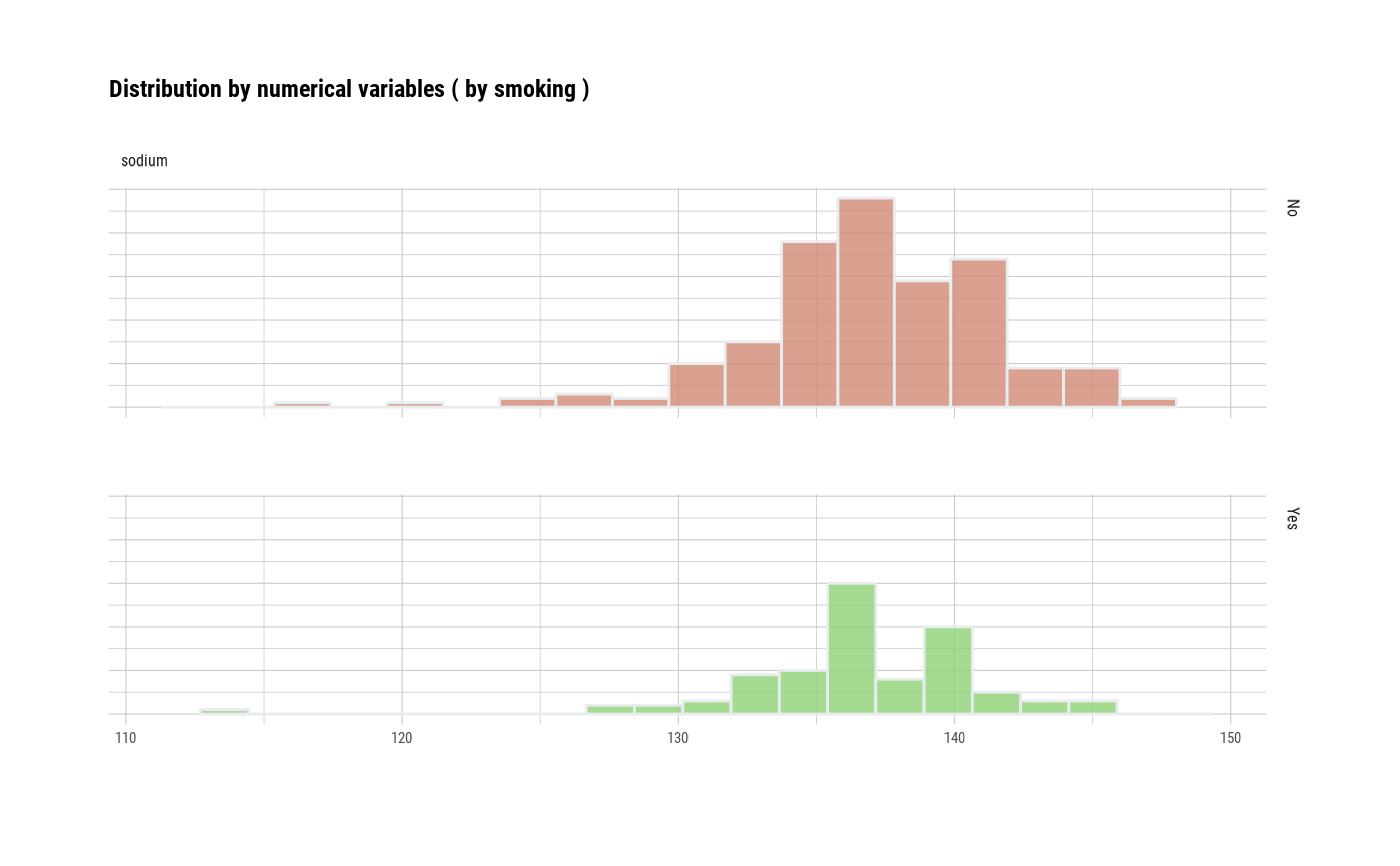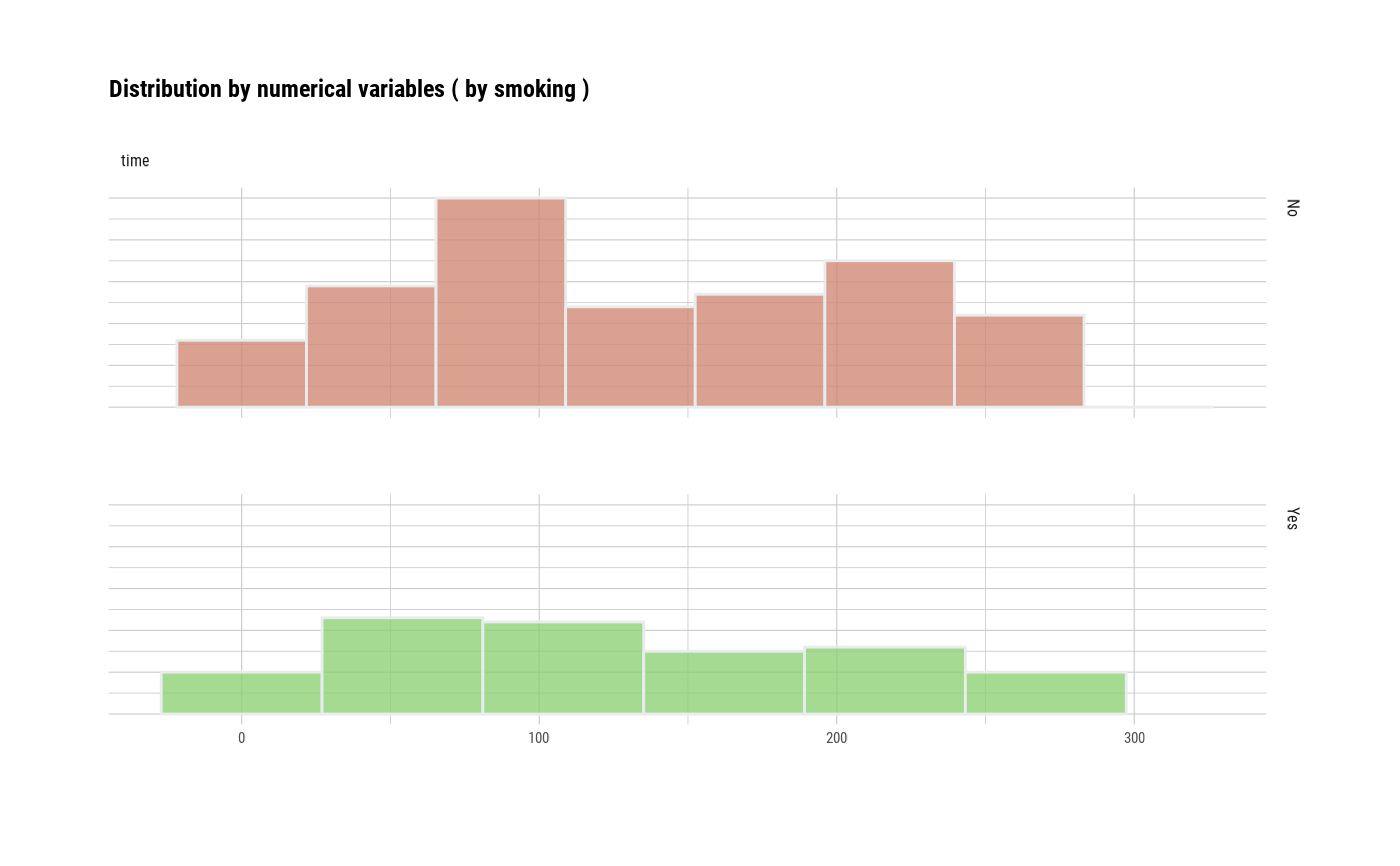# }